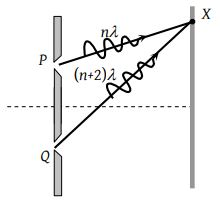Two coherent sources of intensities, I1 and I2 produce an interference pattern. The maximum intensity in the interference pattern will be

(1) I1 + I2

(2) ${I}_{1}^{2}+{I}_{2}^{2}$

(3) (I1 + I2)2

(4) ${\left(\sqrt{{I}_{1}}+\sqrt{{I}_{2}}\right)}^{2}$

Concept Questions :-

Superposition principle
High Yielding Test Series + Question Bank - NEET 2020

Difficulty Level:

Two beams of light having intensities I and 4I interfere to produce a fringe pattern on a screen. The phase difference between the beams is $\frac{\pi }{2}$ at point A and π at point B. Then the difference between the resultant intensities at A and B is

(1) 2I

(2) 4I

(3) 5I

(4) 7I

Concept Questions :-

Superposition principle
High Yielding Test Series + Question Bank - NEET 2020

Difficulty Level:

Two waves are represented by the equations ${y}_{1}=a\mathrm{sin}\omega t$ and ${y}_{2}=a\mathrm{cos}\omega t.$ The first wave

(1) Leads the second by $\pi$

(2) Lags the second by $2\pi$

(3) Leads the second by $\frac{\pi }{2}$

(4) Lags the second by $\frac{\pi }{2}$

Concept Questions :-

Superposition principle
High Yielding Test Series + Question Bank - NEET 2020

Difficulty Level:

If an interference pattern have maximum and minimum intensities in 36 : 1 ratio then what will be the ratio of amplitudes

(1) 5 : 7

(2) 7 : 4

(3) 4 : 7

(4) 7 : 5

Concept Questions :-

Superposition principle
High Yielding Test Series + Question Bank - NEET 2020

Difficulty Level:

In Young's double slit experiment, if the slit widths are in the ratio 1 : 9, then the ratio of the intensity at minima to that at maxima will be

(1) 1

(2) 1/9

(3) 1/4

(4) 1/3

Concept Questions :-

Young Double slit experiment
High Yielding Test Series + Question Bank - NEET 2020

Difficulty Level:

In a certain double slit experimental arrangement interference fringes of width 1.0 mm each are observed when light of wavelength 5000 Å is used. Keeping the set up unaltered, if the source is replaced by another source of wavelength 6000 Å, the fringe width will be

(1) 0.5 mm

(2) 1.0 mm

(3) 1.2 mm

(4) 1.5 mm

Concept Questions :-

Diffraction
High Yielding Test Series + Question Bank - NEET 2020

Difficulty Level:

Two coherent light sources S1 and S2 (λ= 6000 Å) are 1mm apart from each other. The screen is placed at a distance of 25 cm from the sources. The width of the fringes on the screen should be

(1) 0.015 cm

(2) 0.025 cm

(3) 0.010 cm

(4) 0.030 cm

Concept Questions :-

Diffraction
High Yielding Test Series + Question Bank - NEET 2020

Difficulty Level:

The figure shows a double slit experiment P and Q are the slits. The path lengths PX and QX are nλ and (n + 2) λ respectively, where n is a whole number and λ is the wavelength. Taking the central fringe as zero, what is formed at X(1) First bright

(2) First dark

(3) Second bright

(4) Second dark

Concept Questions :-

Young Double slit experiment
High Yielding Test Series + Question Bank - NEET 2020

Difficulty Level:

The Young's experiment is performed with the lights of blue (λ = 4360 Å) and green colour (λ = 5460 Å), If the distance of the 4th fringe from the centre is x, then

(1) x (Blue) = x (Green)

(2) x (Blue)> x (Green)

(3) x (Blue) < x (Green)

(4) $\frac{x\left(Blue\right)}{x\left(Green\right)}=\frac{5460}{4360}$

Concept Questions :-

Young Double slit experiment
High Yielding Test Series + Question Bank - NEET 2020

Difficulty Level:

In Young's double slit experiment, if L is the distance between the slits and the screen upon which interference pattern is observed, x is the average distance between the adjacent fringes and d being the slit separation. The wavelength of light is given by

(1) $\frac{xd}{L}$

(2) $\frac{xL}{d}$

(3) $\frac{Ld}{x}$

(4) $\frac{1}{Ldx}$

Concept Questions :-

Young Double slit experiment